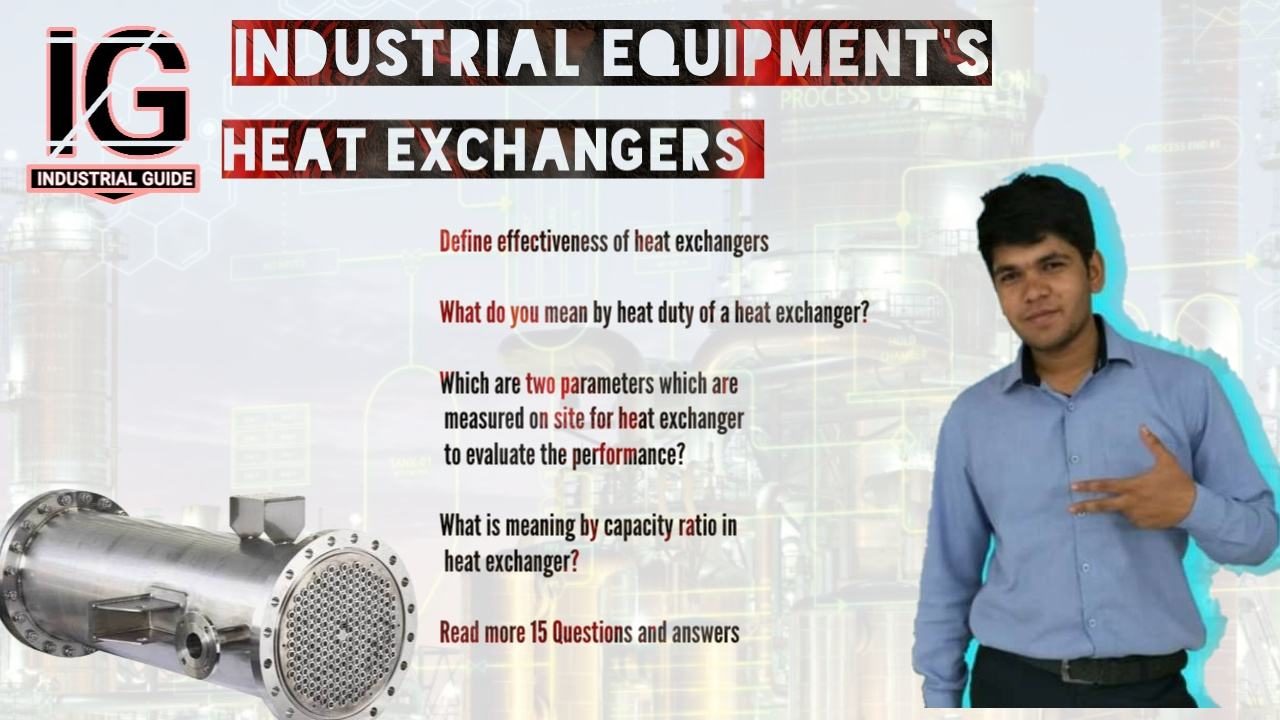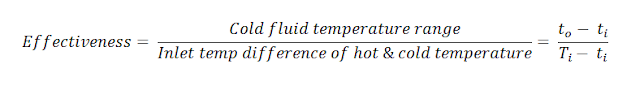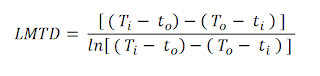## Heat exchangers Interview QuestionsHeat exchangers Interview Questions

### Blog contains

Heat transfer interview questions asked in technical Interviews explained here in easy terms.

### Interview questions contain in this blog

1.  Define effectiveness of heat exchangers
2. What do you mean by heat duty of a heat exchanger?
3. Which are two parameters which are measured on site for heat exchanger to evaluate the performance?
4. What is meaning by capacity ratio in heat exchanger?
5. Find the overall heat transfer co efficient for a shell and tube counter flow heat exchanger.
6. Give the equation to calculate overall heat transfer co efficient?
7. What is LMTD ? Why we use LMTD to calculate overall heat transfer co efficient in shell & tube heat exchanger than arithmetic average?
8. Give the equation to calculate LMTD for a counter flow heat exchanger
9. Give the equation to calculate LMTD for a Parallel flow heat exchanger.
10. Write the equation to calculate heat duty for a heat exchanger.
11. What do you mean by overall heat transfer coefficient?
12. Which factors affecting heat transfer between fluids?
13. What is basis of choosing Shell side & Tube side fluid heat exchanger?

### 1. Define effectiveness of heat exchangers

Heat exchangers effectiveness can be found by

Where
to = Cold water outlet temperature
ti = Cold fluid inlet temperature
Ti = Hot water inlet temperature

### 2. What do you mean by heat duty of a heat exchanger?

Heat duty of a heat exchanger is define as the heat that is transferred from hot fluid to cold fluid per unit time.

###3. Which are two parameters which are measured on site for heat exchanger to evaluate the performance?

1. Hot and cold fluid inlet and outlet temperatures
2. Mass flowrate of cold and hot fluids

### 4. What is meaning by capacity ratio in heat exchanger?

Capacity ratio is

to = Cold water outlet temperature
ti = Cold fluid inlet temperature
Ti = Hot water inlet temperature
To = Hot fluid outlet temperature

### 5. find the over all heat transfer co efficient for a shell and tube counter flow heat exchanger.

Data

Heat exhausted = 11.27 GJ/ hour
Heat transfer area = 96m2
LMTD = 76°C

Heat transfer co efficient can be calculated by following equation

U = Q / ( A * LMTD )
=  11.27 * 10 ^6 / ( 3600 * 96 * 76 )
= 0.43 kW / m2 K

### 6. Give the equation to calculate overall heat transfer co efficient?

Heat exchanger performance is normally evaluated by the overall heat transfer co efficient U that is defined by the equations

U = Qt / ( At* LMTD )

Where is

Q = Heat transferred in kcal/hr
A = Heat transfer surface area in m2
LMTD = Log mean temperature difference in °C
U = Overall heat transfer Coefficient kCal/hr/m2/°C

### 7.  What is LMTD ? Why we use LMTD to calculate overall heat transfer co efficient in shell & tube heat exchanger than arithmatic average?

As we seen heat transfer co efficient
U = Qt / ( At* LMTD )
In heat exchanger hot fluid is losing heat and cold fluid is receiving heat this is not linear.
This is the reason why LMTD is used rather than Trial and error method.

### 8. Give the equation to calculate LMTD for a counter flow heat exchangerWhere
Ti = Hot fluid inlet temperature,
ti = Cold fluid inlet temperature
To = Hot fluid outlet temperature
to = Cold fluid outlet temperature

### 9. Give the equation to calculate LMTD for a Parallel flow heat exchangerWhere
Ti = Hot fluid intet temperature,
ti = Cold fluid inlet temperature
To = Hot fluid outlet temperature
to = Cold fluid outlet temperature

### 10. Write the equation to calculate heat duty for a heat exchanger.

Heat duty Qh = W * Cph * (Ti - To )
Heat duty Qc = W * Cph * (ti - to )

### 11. What do you mean by overall heat transfer coefficient?

The ration of heat flux pr unit difference in approach across heat exchange equipment considering the individual coefficient and heat exchanger metal surface metal conductivity.

The magnitude indicates the ability of heat transfer for a given surface.

Higher the coefficient lesser will be the heat transfer surface requirement.

### 12. Which factors affecting heat transfer between fluids?

#### 1. Types of fluid

Gas can transfer more heat comparison to liquid, and liquids can transfer more heat comparison to solid.

#### 2. Types of material

Conductive material can easily transfer heat in comparison of resistivity material & Non metals.

#### 3. Thickness of material

Equipment's with less thickness can easily transfer against higher thickness material.

#### D. Surface area

Heat transfer rate is directly proportional to surface material.
More active surface area more heat transfer.
Fourier's law
Q = - k A dt/dx

#### E. Liquid pressure

Liquids with high pressure can transfer more heat.
In high pressure molecules of fluid is more closer so vibration of heat can easily transfer atom to atom.

#### F. Turbulence of fluid

Fluid turbulence is directly proportional to turbulence of fluid.
Turbulence flow has high tendency of transfer heat than laminar flow.
This is the reason baffles are provided in heat exchanger.

#### G. Temperature difference between fluids.

High Temperature difference between two fluid can transfer more heat.

#### H. Thermal conductivity of equipment material of construction [MOC]

Each metal has unique conductivity to transfer heat. Copper has high thermal conductivity then Iron So, Copper can easily heat respect to Iron.

#### I. Velocity of fluids in heat exchanger

High velocity fluids prevent scaling and chocking in heat transfer equipments so more heat transfer takes place in high velocity fluids.

#### J.Direction of Flow of fluids in exchange.

There are three flow patterns
Co current flow - Both fluids flowing in parallel stream
Split flow - Both fluids flow in right angle
Counter current flow - Both fluids are flowing in opposite direction

Heat transfer rate in fluids patterns
Co current flow < Split flow < Counter current flow

#### L.Amount of liquids

Heat exchanger gives more output when more amount of flow given.

### 13. What is basis of choosing Shell side & Tube side fluid heat exchanger?

If any Phase change from liquid to gas occur like Vaporization or Condensation then it is selected on shell side because Shell side sufficient space which prevents layer building of Condensation which provides additional resistance.

Heat exchange with both side phase change like in Reboilers in this cases other factors also considered.
Now we will see Fluid allocation if phase change is not there.

Factors on which basis fluid allocation done
1. Corrosive fluid
2. Fouling
3. Fluid Temperature
4. Operating Pressure
5. Fluid flow rates and viscosity

#### 1. Corrosive fluid

Corrosive fluid is allowed in tube side.

Reason -
• There are costly parts in shell side like Tube sheet, Baffles, Tubes, etc.
• Tubes can be easily replaceable

#### 2. Fouling

Heat exchangers tubes are easily cleanable so liquids with high fouling tendency means dirty fluid allocated to tube side

#### 3. Fluid Temperature

Special alloys required for handling high temperature fluid.
High temperature and low temperature fluids are flowed in tube side
Reason - When too hot or cold fluid selected on shell side energy is loss in atmosphere. Heat loss may avoided with insulation.

#### 4. Operating pressure

Fluid with high pressure selected on tube side.
Reason - If any damage happens then tubes can be easily changed.

#### 5. Fluid flow rates & Viscosity of fluid.

Reynolds number states that Low flow rates with high viscosity has low reynolds number. Low reynolds number means low heat transfer.
If we select it to Shell side then heat transfer can be efficient [Baffles increases heat transfer]